• ## 特征提取算法

千次阅读 2019-06-15 16:27:59
特征提取算法1、SIFT（1）构建高斯差分金字塔（2）特征点的搜索尺度空间极值点搜索子像元插值删除边缘效应2、Harris角点 1、SIFT （1）构建高斯差分金字塔 高斯滤波是实现尺度变换的唯一变换核。 I(x,y)I(x,y)I(x,y...
特征提取1、背景2、边界预处理Moore boundary tracing algorithmChain CodesFreeman Chain Codesslope chain codes (SCCs)minimum-perimeter polygon (MPP)signaturesskeletons3、Region Feature Descriptors3.1 区域描述子和特征向量3.2 Topological Descriptors3.3 Texturestatistical approaches： 利用灰度直方图的统计矩statistical approaches： 利用灰度灰度共生矩阵spectral approaches： 基于傅里叶频谱LBP特征3.4 Moment Invariants4、Principal Components as Feature DescriptorsHotelling Transform (principal components transform)使用主成分对尺寸、平移、旋转变化进行归一化1、SIFT（1）构建高斯差分金字塔（2）特征点的搜索尺度空间极值点检测（关键点的初步探查）子像元插值删除边缘效应2、Harris角点检测算子3、HOG
1、背景
特征是一种在计算机视觉和图像处理中用来进行物体检测的特征描述子。
特征提取（feature extraction）包括两部分：特征检测（feature detection）和特征描述（feature description）。假设我们使用object corners 作为特征，检测就是在一个区域或图像中寻找corners；描述就是给检测到的特征赋予定量属性（quantitative attributes），如 corner orientation和location。
特征是用来区分对象的，一般要independent于location, rotation, 和 scale，另外独立于illumination levels 和 changes caused by the viewpoint between the imaging sensor and the scene 也很重要。（平移不变性、旋转不变性、尺度不变性、光照不变性、视点不变性）
上面说的"independent"一词通常具有两种含义之一：invariant或covariant。特征描述子是不变的，即对于一系列的图像变换，其值保持不变；特征描述子是协变的，即对于一系列变换，应用任意一个变换会导致特征描述子发生相同的变换。通过协变量的规范化（normalize）可以转换为不变量，例如对于旋转是协变的，我们可以通过将特征描述子旋转至预定义的方向，这样旋转协变的特征描述子就转换为了旋转不变的也正描述子。
特征分为局部特征（local feature description）和全局特征（global）。如果一个特征适用于一个集合中的一个成员，则称这个特征是局部的；如果一个特征适用于整个集合，则称这个特征是全局的。“集合”和“成员”视具体任务而定。
特征提取算法通常会产生多个特征描述值，将这些数值特征打包称之为一个特征向量（feature vector），其元素都是描述子（descriptors）。如果采用n个descriptors，则特征向量是n维的，则包含所有特征向量的空间是一个n维特征空间（feature space）。
在特征提取之前应使用预处理操作对输入图像进行归一化（normalize），例如直方图均衡化或规定化（histogram equalization or specification），可以用于部分弥补光照变化的影响。
2、边界预处理
Moore boundary tracing algorithm
假设一幅二值图像，物体区域的像素值为1，背景区域像素为0，Moore边界跟踪算法流程如下：

从左到右从上到下依次扫描图像，找到第一个像素值为1的点，作为起始点，记为$b_0$，将该点左边点记为$c_0$。
令$b=b_0$，$c=c_0$，从$c$开始以顺时针方向扫描$b$的八邻域像素点，扫描过的序列依次记为$n_1,...,n_8$，假设序列中第一个像素值为1的点为$n_k$。
令$b=n_k$，$c=n_{k-1}$，从$c$开始以顺时针方向扫描$b$的八邻域像素点，扫描过的序列依次记为$n_1,...,n_8$，假设序列中第一个像素值为1的点为$n_k$。
重复第三步，直至$b=b_0$。算法停止时所有$b$点组成的序列是边界点的有序集合。

Chain Codes
链码通过指定长度和方向的直线段的连接序列来表示边界。
Freeman Chain Codes
定义四方向和八方向链码各个方向对应的数字。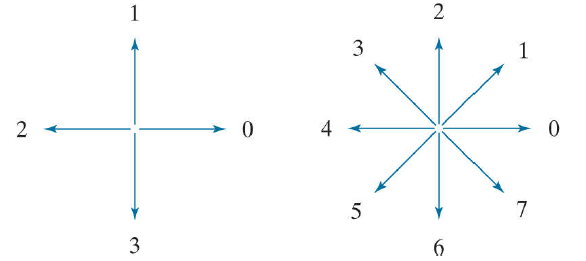在实际使用中，不需要那么精细的链码（一是太长，二是些微扰动对边界形状影响很大），因此首先利用更大的网格间距对边界进行重采样，使得多个像素点落在一个网格内，根据原始像素点与网格结点的距离确定粗网格的边界点。然后以最左最上的边界点为起始点，顺时针方向按照以上的四方向或八方向数字定义对边界点进行数字编码。

链码可以相对于起点进行规范化（即对于任意起点，规范化的链码都相同）：将链码视为一个循环序列，并重新定义起点使得序列对应的数最小。
链码可以通过其一阶差分（first difference）对旋转进行规范化（即对边界进行任意方向旋转，规范化的链码都相同）。
例如对于四方向链码10103322，我们先将其相对于起点规范化，得到链码01033221，其一阶差分的第一个数为最后一个数与起点相隔的方向数为3（因为最后一个数1要逆时针转动3次转到起点0），如图所示，一阶差分为3133030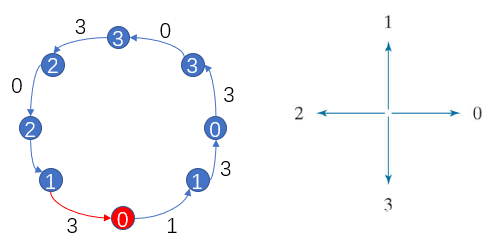尺度规范化（Size normalization）可以通过改变重采样网格间距实现。

slope chain codes (SCCs)
SCCs 通过用将某条固定长度线段的两个端点置于曲线上并遍历曲线得到，用一个斜率变化序列来表示，取值范围为(-1,1)，可以用来近似曲线。SCCs独立于旋转、平移，并且可以对尺度变化进行规范化。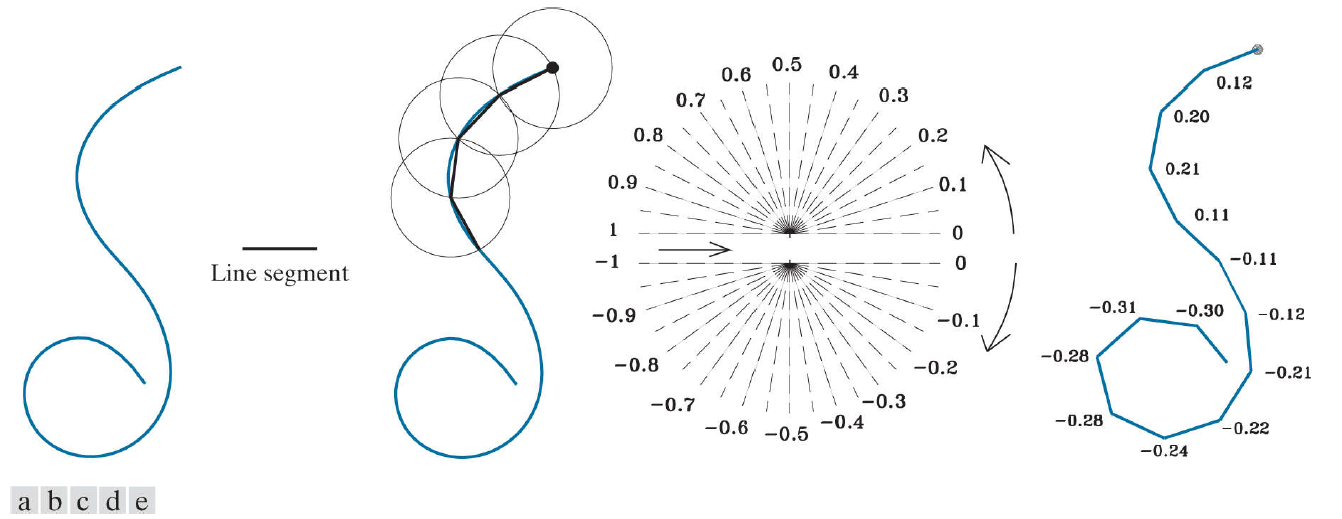minimum-perimeter polygon (MPP)
可以用边界的最小周长多边形（MPP）来近似表示边界。我们首先用一系列的cell 包围边界，称为 a cellular complex。则边界的MPP的顶点要么是 the cellular complex 内壁的凸顶点，要么是其内壁凹顶点对应的在外壁的镜像凹顶点。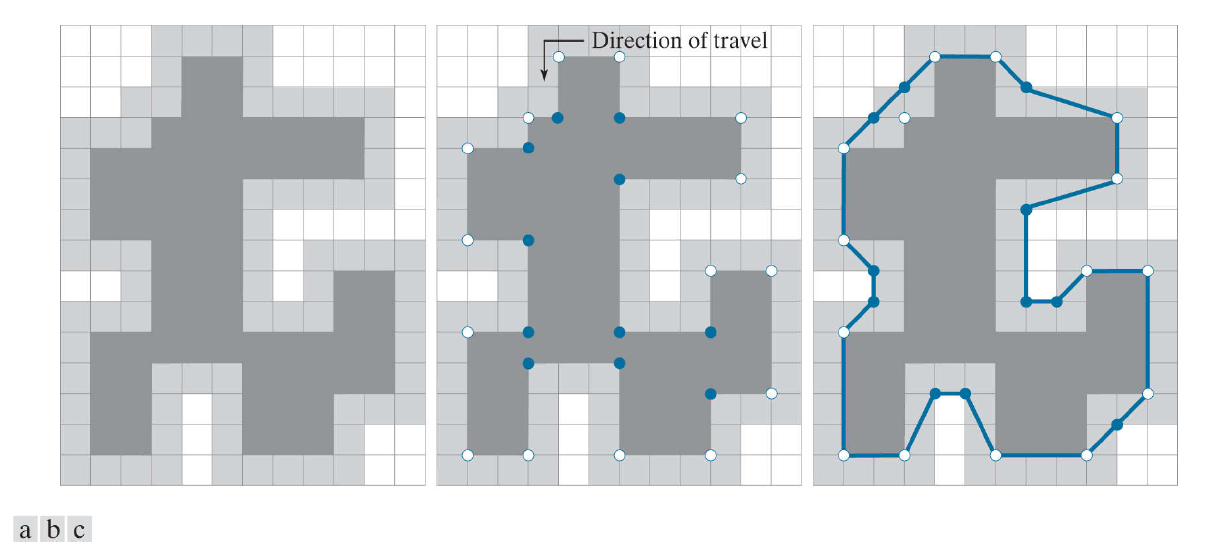MPP算法通过遍历边界所有的顶点来判断该顶点是否为MPP的顶点。边界的顶点可以采用边界跟踪算法生成。
MPP可以用来近似表示边界，能大大减小数据量，此外对边界具有平滑效果（视网格大小而定）。
signatures
a signature 是用一个一维函数表示二维边界。
skeletons
边界(boundaries)和骨架(skeletons)都可以用来描述区域形状。骨架可以通过两种方法获得：基于形态学的腐蚀变薄处理；基于距离变换获得区域中轴的方法。
3、Region Feature Descriptors
3.1 区域描述子和特征向量

The major and minor axes of a region

The bounding box of a region

The area of a region $A$：the number of pixels in the region

The perimeter of a region $p$：the length of its boundary

compactness(dimensionless measure)：$compactness=\frac{p^2}{A}$

circularity/roundness(dimensionless measure)：$circularity=\frac{4\pi A}{p^2}$
（$compactness$和$circularity$ are independent of size, orientation, and translation.）

The effective diameter：$d_e=2\sqrt{\frac{A}{\pi}}$ (independent of orientation and translation)

The eccentricity of a region：等于与该区域具有相同二阶中心矩的椭圆的离心率
（ $椭圆离心率=\frac{c}{a}=\frac{\sqrt{a^2-b^2}}{a}=\sqrt{1-(\frac{b}{a})^2}$    (a>=b)  即椭圆中心到焦点的距离与椭圆中心到主轴端点距离的比值）
（给定一个区域，我们令椭圆的主轴和副轴分别与区域的轴对齐，获得该区域的近似椭圆。定义协方差矩阵$C$：$C=\frac{1}{K-1} \sum^K_{k=1}(z_k-\overline{z})(z_k-\overline{z})^T$。其中$z_k$是一个二维向量，其元素为区域中像素点的坐标；$K$为像素点个数；$\overline{z}$是均值向量。主轴和副轴长度的一半$a$和$b$分别为协方差矩阵的最大值和最小值，主轴和副轴的方向分别为特征值对应的特征向量方向。 另外有结论：圆的离心率为0；直线的离心率为1。离心率的范围为[0,1]。）

通常一个描述子无法区分个体。我们将多个描述子组合成一个特征向量，所有这样的特征向量构成特征空间。 在特征空间中，我们更易看出个体间的相似性和差异性。
3.2 Topological Descriptors
Topology：研究图形不受任何变形影响（如拉伸、旋转）的特性，假设不存在撕裂（no tearing）和折叠的情况。(例如：区域空洞的个数)

The Euler number $E$ of a region：$E=C-H$。（the number of holes $H$, and connected components $C$）
Euler formular：$V-Q+F=C-H$。（对于由线段组成的区域（如多边形网络），denoting the number of vertices by $V$, the number of edges by $Q$, and the number of faces by $F$，有Euler formular。）

3.3 Texture
一般描述区域的纹理可以通过：smoothness, coarseness, and regularity …

statistical approaches for describing the texture of a region：smooth. coarse, grainy …
spectral approaches：detect global periodicity

statistical approaches： 利用灰度直方图的统计矩
设$z$是表示灰度的随机变量，$p(z_i), i=0,1,...,L-1$ 为对应的归一化直方图，则$z$的$n$阶矩为：
$\mu_n(z)=\sum^{L-1}_{i=0} (z_i - m)^n p(z_i)$
其中，$m=\sum^{L-1}_{i=0}z_i p(z_i)$。
可以发现，

$\mu_0=1, \mu_1=0$。
二阶矩$\mu_2=\sigma^2(z)$是比较重要的纹理描述子。（It’s a measure of intensity contrast that can be used to establish descriptors of relative intensity smoothness：$R(z)=1-\frac{1}{1+\frac{\sigma^2(z)}{(L-1)^2}}$。 常亮度区域$R=0$。方差越大的区域$R$越接近1。）
the third moment $\mu_3(z)$ is a measure of the skewness of the histogram.
the fourth moment $\mu_4(z)$ is a measure of its relative flatness.
uniformity：$U(z)=\sum^{L-1}_{i=0} p^2(z_i)$。（常数区域，$U$最大）
average entropy：$e(z)=-\sum^{L-1}_{i=0}p(z_i)\log_2 p(z_i)$。（常数区域，$e=0$）

总结：

mean 反映整体亮度水平，但不反映纹理，一般不作为纹理描述子
standard deviation 和 $R$ 一样，反映纹理的光滑性，值越小表明越光滑（常数区域值为0）
third moment 反映了直方图的对称性，小于0表示直方图偏左，大于0表示偏右，即表示整体图像亮度偏暗或偏亮
uniformity 均匀性。粗纹理对应于低值，值越大表明图像越光滑
entropy 不确定性。随着uniformity的减小而增大，值越小，表明亮度变化越小，图像越光滑

statistical approaches： 利用灰度灰度共生矩阵
仅利用直方图计算的纹理描述子不包含像素的空间位置信息。为了引入空间位置信息，采取graylevel co-occurrence matrix。
定义灰度共生矩阵： 假设$Q$是位置算子，co-occurrence matrix $G$ is a matrix whose element $g_{ij}$ is the number of times that pixel pairs with intensities $z_i$ and $z_j$ occur in image $f$ in the position specified by $Q$。（即灰度共生矩阵$G$的元素$g_{ij}$表示像素$z_i$和$z_j$在满足$Q$指定的位置关系下在图像$f$中出现的次数。）
The quantity $p_{ij}=\frac{g_{ij}}{n}$ is an estimate of the probability that a pair of points satisfying $Q$ will have values $(z_i,z_j)$。
基于共生矩阵的纹理描述子：

maximum probability： $\max \limits_{i,j} (p_{ij})$

spectral approaches： 基于傅里叶频谱
傅里叶频谱的特征可以用作纹理描述（周期性纹理）。
LBP特征
LBP（Local Binary Pattern, 局部二值模式）是一种用来描述图像局部纹理特征的算子。
（1）首先将图像划分为16×16的小区域（cell）；
（2）对每个cell中的每个像素，将相邻的8个像素的灰度值与之比较，若大于该灰度值，则标记为1，否则标记为0。33邻域内的8个点经比较可产生8位二进制数，即为该像素点的LBP值。
（3）将二进制LBP值转换为十进制，计算每个cell的LBP直方图，即统计每个LBP值出现的频率，并对直方图进行归一化。
（4）将1616个cell的直方图进行连接得到一个特征向量，即整幅图的LBP纹理特征向量。
然后便可利用SVM或者其他机器学习算法进行分类了。
应用：纹理特征提取，提取的特征是图像的局部纹理特征
3.4 Moment Invariants
The 2-D moment of order $(p+q)$ of an $M×N$ digital image $f(x,y)$：
$m_{pq}=\sum^{M-1}_{x=0} \sum^{N-1}_{y=0} x^p y^q f(x,y)$
The corresponding central moment of order $(p+q)$：
$\mu_{pq}=\sum^{M-1}_{x=0} \sum^{N-1}_{y=0} (x-\overline x)^p (y-\overline y)^q f(x,y)$
其中，$\overline x=\frac{m_{10}}{m_{00}}$，$\overline y=\frac{m_{01}}{m_{00}}$。
The normalized central moment of order $(p+q)$：
$\eta_{pq}=\frac{\mu_{pq}}{\mu^{\gamma}_{00}}$
其中，$\gamma=\frac{p+q}{2}+1$ for $p+q=2,3,...$
一组共7个2-D moment invariants can be derived from the second and third normalized central moments：$\phi_1, \phi_2, ..., \phi_7$。这组矩对于平移、尺度变化、镜像、旋转都是不变的（invariant）。
4、Principal Components as Feature Descriptors
假设有$n$幅图像，对于任一位置，这$n$幅图像对应位置的像素组成一个$n$维列向量：
$x=\left( \begin{array}{c} x_1 \\ x_2 \\ ... \\ x_n \end{array} \right)$
我们将$x$视为一个随机向量，并定义均值向量和协方差矩阵：
$m_x=E\{x\}$
$C_x=E\{ (x-m_x)(x-m_x)^T \}$
由于$x$是一个$n$维列向量，则$C_x$是一个$n×n$矩阵。$C_{ii}$表示向量$x$的第$i$个分量的方差，$C_{ij}$表示向量$x$中的$x_i$与$x_j$的协方差。如果$x_i$和$x_j$不相关，则$C_{ij}=0$。
$C_x$是一个实对称矩阵，总可以找到$n$个正交的特征向量。令矩阵$A$的每一行为$C_x$的特征向量，且对应的特征值按从大到小排序（即$A$的第一行是最大特征值对应的特征向量）。
Hotelling Transform (principal components transform)
作变换：
$y=A(x-m_x)$
有性质：$m_y=0$，$C_y=ACA^T$。$C_y$是一个对角矩阵，对角线上的元素为其特征值按从大到小排序。因此向量$y$的各个分量互不相关。$C_x$和$C_y$具有相同的特征值。
通过$y$可以重建$x$： ($A$为正交矩阵，$A^T=A^{-1}$)
$x=A^T y +m_x$
假设$A_k$是由$k$个最大特征值对应的特征向量组成的，$A_k$的维度为$k×n$，作变换：
$y_k=A_k(x-m_x)$
(可以发现 $x_{n×1}$ --> $y_{k×1}$ 实现了降维。)
利用$y_k$我们获得$x$的近似：
$\hat x =A^T_{k} y + m_x$
$x$与$\hat x$的均方误差为：$e_{ms}=\sum^{n}_{j=k+1} \lambda_j$。
使用主成分对尺寸、平移、旋转变化进行归一化
假设有一个物体，我们记物体像素点的坐标为$x=(x_1,x_2)^T$，物体区域的所有像素点构成数据总体，求其均值向量和协方差矩阵，然后对这个物体区域实行主成分变换。式子$y=A(x-x_m)$做了两件事：（1）$x-x_m$将变换后的原点确定为物体的质心；（2）变换后的y坐标向量是x的旋转，即将坐标轴与两个特征向量的方向对齐。
1、SIFT
尺度不变特征转换（Scale-invariant feature transform 或SIFT）用于检测和描述图像的局部性特征，它在空间尺度中寻找极值点，并提取出位置、尺度、旋转不变量，对于光线、噪声以及微小视角改变的容忍度较高。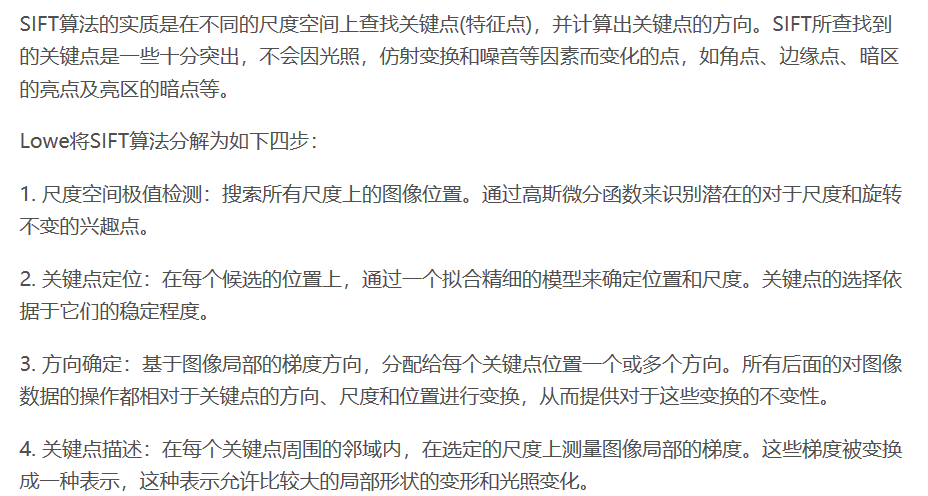（1）构建高斯差分金字塔
高斯滤波是实现尺度变换的唯一变换核。

$I(x,y)$表示原图像。
$G(x,y,\sigma)$表示高斯滤波器，其中$G(x,y,\sigma)=\frac{1}{2\pi\sigma^2}e^{-\frac{x^2+y^2}{2\sigma^2}}$。
$L(x,y,\sigma)=G(x,y,\sigma)\otimes I(x,y)$。一系列的$\sigma_i$可以生成一系列的$L(x,y,\sigma_i)$图像。这一系列图像称为原图像的一个尺度空间表示。
$DoG$表示高斯差分（Difference of Gaussians）。$D(x,y,\sigma)=(G(x,y,k\sigma)-G(x,y,\sigma))\otimes I(x,y)=L(x,y,k\sigma)-L(x,y,\sigma)$。

使用高斯差分函数的原因：尺度归一化的高斯拉普拉斯函数$\sigma^2 \nabla^2 G$的极大值和极小值相比其他特征提取函数，如梯度、Hessian 或Harris角特征，能够产生最稳定的图像特征。而高斯差分函数近似于尺度归一化的高斯拉普拉斯函数。
在实际计算中，使用高斯金字塔每组中相邻上下两层图像相减，得到高斯差分图像。然后进行极值检测。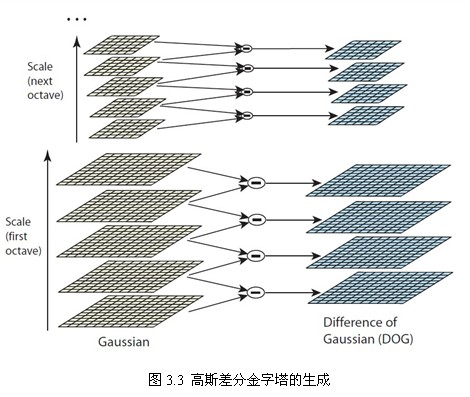（2）特征点的搜索
尺度空间极值点检测（关键点的初步探查）
为了在高斯差分金字塔中寻找尺度空间的极值点，每个采样点要和它所有的相邻点进行比较，包括图像域和尺度域。即对于任意一个检测点要和它同尺度的8个相邻点以及上下相邻尺度的9*2个点共26个点比较。搜索过程从每组的第二层开始，以第二层为当前层，对第二层的DoG图像中的每个点取一个3×3的立方体，立方体上下层为第一层与第三层。这样搜索得到的极值点既有位置坐标又有空间尺度坐标。当S=3时，每组要搜索3层。
这样产生的极值点并不全是稳定的特征点，因为某些极值点响应较弱，而且DoG算子会产生较强的边缘响应。
子像元插值
上面的尺度空间极值点的搜索是在离散空间中进行的，检测到的极值点并不是真正意义上的极值点。需要利用已知的离散空间点插值到连续空间极值点，即子像元插值。
通过多次迭代得到最终候选点的精确位置与尺度，将其代入公式求得$D(x)$，并求其绝对值。如果其绝对值低于阈值的将被删除。
删除边缘效应
选取$\gamma=10$，即主曲率比值大于10的特征点将被删除。
参考资料：
 SIFT定位算法关键步骤的说明
2、Harris角点检测算子
Harris角点检测算子具有旋转不变性和尺度不变性，且对亮度和对比度变化不敏感。
若某个窗口内的图像存在角点，则在各个方向上移动这个特征的小窗口，窗口内区域的灰度会发生较大的变化。因此对于图像$I(x,y)$，定义点$(x,y)$平移$(\Delta x, \Delta y)$后的自相似性，即自相关函数：
$c(x,y;\Delta x,\Delta y)=\sum_{w(u,v) \in W(x,y)} w(u,v)(I(u,v)-I(u+\Delta x, v+\Delta y))^2$
该函数可近似为二项函数：
$c(x,y;\Delta x, \Delta y) \approx A \Delta x^2 + 2C \Delta x \Delta y + B \Delta y^2 = \left[\Delta x, \Delta y \right] M(x,y)\left[ \begin{array}{c}\Delta x \\ \Delta y\end{array} \right]$
其中，
$A=\sum_w I^2_x, B=\sum_w I^2_y, C=\sum_w I_x I_y, M(x,y)=\left[ \begin{array}{cc}A & C \\ C & B \end{array} \right]$
当$M$的两个特征值都大且近似相等时，存在角点。我们可以通过计算一个角点响应值$R$来判断角点。
$R=detM-\alpha(traceM)^2$
其中, $\alpha$的取值范围为$0.04 \thicksim 0.06$，
$det M=\lambda_1 \lambda_2=AC-B^2$
$trace M=\lambda_1+\lambda_2=A+C$
算法流程如下：

（1）计算图像$I(x,y)$在$X$和$Y$两个方向的梯度$I_x$、$I_y$。
（2）计算图像两个方向上梯度的乘积$I^2_x$、$I^2_y$和$I_{xy}=I_x I_y$。
（3）进行高斯加权，生成矩阵$M$的元素$A$、$B$和$C$。
$A=g(I^2_x)=I^2_x \otimes w, C=g(I^2_y)=I^2_y \otimes w, B=g(I_{xy})=I_{xy} \otimes w$
（4）计算每个像素的Harris响应值$R$，并对小于某一阈值$t$的$R$置为0。
（5）在3×3或5×5的邻域内进行非极大值抑制，局部最大值即为图像中的角点。

3、HOG
HOG(Histogram of Oriented Gradient，方向梯度直方图)用于描述图像局部纹理的特征。HOG特征通过激素那和统计图像局部区域的方向梯度直方图来构成特征。
思想：在一幅图像中，局部目标的表象和形状能够被梯度或边缘的方向密度分布很好地描述。其本质为：梯度的统计信息，而梯度主要存在于边缘的地方。
算法步骤：
（1）灰度化和Gamma校正：能够有效降低阴影和光照变化的影响。
（2）计算梯度的幅值和方向：
（3）构建方向的直方图：将图像分为若干个cell。假设每个cell为6*6个像素，假设采用9个bin的方向直方图来统计梯度信息（即将360度分为9个方向块，第一个bin是0~40°，第2个bin是40-80° ）。假设某个像素梯度大小为2，方向为30°，则梯度方向直方图的第一个bin计数加2（即梯度大小用来作权值，用双线性内插法将幅值累加到直方图中?）。这样每个cell都能计算出一个梯度方向直方图，即对应的特征向量（上述为9维的特征向量）。
（4）把小cell组合成更大的block，block内归一化梯度直方图：将小cell组合成大的、空间上连通的blocks。一个block内所有cell的特征向量串联起来便得到该block的HOG特征（归一化去除光照和阴影的影响）。（注意，这些blocks是有重叠的，即每个cell的特征会以不同的结果多次出现在最后的特征向量中，我们将归一化后的block描述符称为HOG描述符。）
（5）收集所有blocks的特征，并将它们结合成最终的特征向量供分类使用。
应用：HOG+SVM进行行人检测
参考资料：
Harris角点
图像特征提取三大法宝：HOG特征，LBP特征，Haar特征


展开全文• SIFT特征提取算法实验 SIFT特征提取算法 1.1 算法定义 1.2 SIFT特征提取算法实验： 1.3 构造数据集 检测提取感兴趣点 2.1代码实现： 2.2.绘制特征点代码分析： 提取数据集中每张图片的SIFT特征并展示 3.1实现对比： ...
• 常见的特征提取算法主要分为以下3类： 基于颜色特征：如颜色直方图、颜色集、颜色矩、颜色聚合向量等； 基于纹理特征：如Tamura纹理特征、自回归纹理模型、Gabor变换、小波变换、MPEG7边缘直方图等； 基于形状...

模式识别中进行匹配识别或者分类器分类识别时，判断的依据就是图像特征。用提取的特征表示整幅图像内容，根据特征匹配或者分类图像目标。
常见的特征提取算法主要分为以下3类：
基于颜色特征：如颜色直方图、颜色集、颜色矩、颜色聚合向量等；
基于纹理特征：如Tamura纹理特征、自回归纹理模型、Gabor变换、小波变换、MPEG7边缘直方图等；
基于形状特征：如傅立叶形状描述符、不变矩、小波轮廓描述符等；
LBP特征提取算法
LBP（Local Binary Patterns，局部二值模式）是提取局部特征作为判别依据的，一种有效的纹理描述算子，度量和提取图像局部的纹理信息，它具有旋转不变性和灰度不变性等显著的优点，对光照具有不变性。用于纹理特征提取。而且，提取的特征是图像的局部的纹理特征。有多种改进型，LBP结合BP神经网络已经用于人脸识别等领域。

灰度不变性：指的是光照变化是否会对描述产生影响。以上面的8邻域来说，光照变化很难改变中心像素点的灰度值与周围8个像素的大小关系。因为光照是一种区域性质的变化，而不是单像素性质的变化。

1、LBP特征描述
LBP的基本思想是定义于像素的8邻域中（3x3的窗口）, 以中心像素的灰度值为阈值, 将周围8 个像素的值与其比较, 如果周围的像素值小于中心像素的灰度值, 该像素位置就被标记为0, 否则标记为1。这样3x3的邻域内的八个点经过比较能够产生8位二进制数（通常转换为十进制，即LBP码，共256种），每个像素得到一个二进制组合, 即得到该窗口中心像素点的LBP值，并用这个值来反映该区域的纹理信息。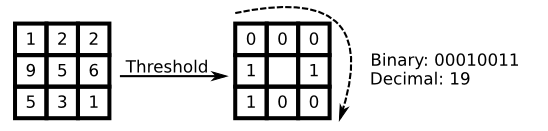2、LBP的改进版本：
（1）圆形LBP算子
基本的 LBP算子的最大缺陷在于它只覆盖了一个固定半径范围内的小区域，这显然不能满足不同尺寸和频率纹理的需要。为了适应不同尺寸的纹理特征，达到灰度和旋转不变性的要求。将 3×3邻域扩展到任意邻域，并用圆形邻域代替了正方形邻域，改进后的 LBP 算子允许在半径为 R的圆形邻域内有任意多个像素点。从而得到了诸如半径为R的圆形区域内含有P个采样点的LBP算子。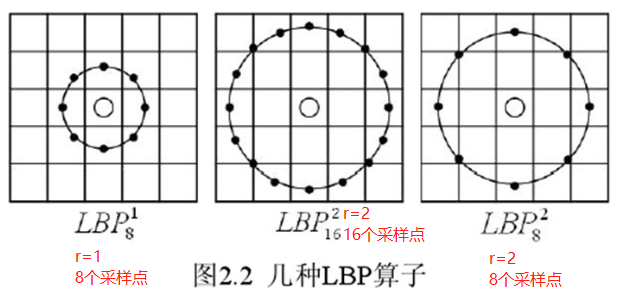（2）LBP旋转不变模式
从 LBP 的定义可以看出，LBP 算子是灰度不变的，但却不是旋转不变的。图像的旋转就会得到不同的LBP值。
Maenpaa等人又将 LBP算子进行了扩展，提出了具有旋转不变性的 LBP 算子，即不断旋转圆形邻域得到一系列初始定义的 LBP值，取其最小值作为该邻域的 LBP 值。
下图给出了求取旋转不变的 LBP 的过程示意图，图中算子下方的数字表示该算子对应的 LBP值，图中所示的 8 种 LBP模式，经过旋转不变的处理，最终得到的具有旋转不变性的 LBP值为 15。也就是说，图中的 8种 LBP 模式对应的旋转不变的 LBP模式都是 00001111。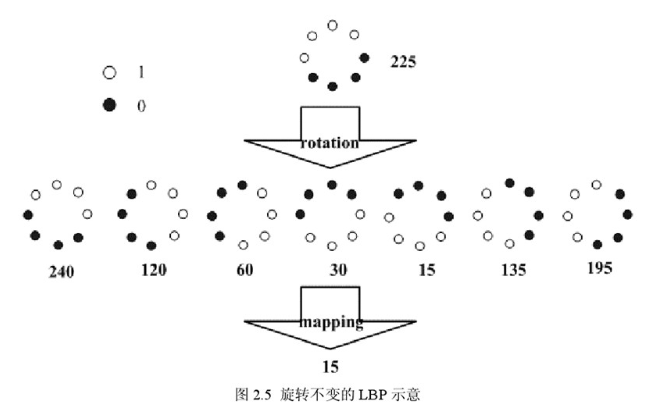（3）LBP等价模式
一个LBP算子可以产生不同的二进制模式，对于半径为R的圆形区域内含有P个采样点的LBP算子将会产生2p种模式。很显然，随着邻域集内采样点数的增加，二进制模式的种类是急剧增加的。例如：5×5邻域内20个采样点，有220＝1,048,576种二进制模式。
如此多的二值模式无论对于纹理的提取还是对于纹理的识别、分类及信息的存取都是不利的。同时，过多的模式种类对于纹理的表达是不利的。例如，将LBP算子用于纹理分类或人脸识别时，常采用LBP模式的统计直方图来表达图像的信息，而较多的模式种类将使得数据量过大，且直方图过于稀疏。因此，需要对原始的LBP模式进行降维，使得数据量减少的情况下能最好的代表图像的信息。
为了解决二进制模式过多的问题，提高统计性，Ojala提出了采用一种“等价模式”（Uniform Pattern）来对LBP算子的模式种类进行降维。Ojala等认为，在实际图像中，绝大多数LBP模式最多只包含两次从1到0或从0到1的跳变。因此，Ojala将“等价模式”定义为：当某个LBP所对应的循环二进制数从0到1或从1到0最多有两次跳变时，该LBP所对应的二进制就称为一个等价模式类，除了等价模式以外的模式都归一一类，称为混合模式类。计算跳变的方法：如10010111，首先第一二位10，由1—>0跳变一次；第二、三位00，没有跳变；第三、四位01，由0—>1跳变一次，第四、五位10，由1—>0跳变一次；第五六位01，由0—>1跳变一次；第六七位11，没有跳变；第七八位11，没有跳变；第八位和第一位11，没有跳变；故总共跳变4次。
通过这种改进，二进制模式的种类大大减少，而不会丢失任何信息，模式种类由原来的2^p减少为p*(p-1)+2种。对于3×3邻域内8个采样点来说，二进制模式由原始的256种减少为58种，这使得特征向量的维数更少，并且可以减少高频噪声带来的影响。

1、0次跳变，只有两种，即000..或者1111....
2、1次跳变，两种可能，0—>1或者1—>0：
（1）0开头：0...01、0....011，一共有p-1种；
（2）1开头：1...10、1...100、1..1000、等等，一种有p-1种；
综合，一次跳变有2（p-1）种。
2、两次跳变，0—>1—>0和1—>0—>1
0—>1—>0：第一次跳变位置在第2、第3、........p-1位置时，对应的第二次跳变有p-2种、p-3种....1种的跳变位置，所以共有(p-1)(p-2)/2种模式；
1—>0—>1：相应的也有(p-1)(p-2)/2种；
综合有(p-1)(p-2)种模式
综上一共有2+2(p-1)+(p-1)(p-2)=p(p-1)+2种模式可能。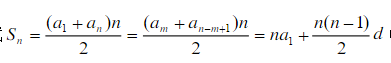等价模式代表了图像的边缘、斑点、角点等关键模式，等价模式占了总模式中的绝大多数，所以极大的降低了特征维度。利用这些等价模式和混合模式类直方图，能够更好地提取图像的本质特征。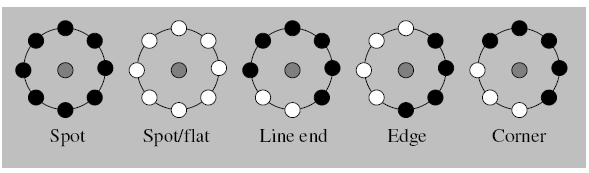3、LBP特征用于检测的原理
显而易见的是，上述提取的LBP算子在每个像素点都可以得到一个LBP“编码”，那么，对一幅图像（记录的是每个像素点的灰度值）提取其原始的LBP算子之后，得到的原始LBP特征依然是“一幅图片”（记录的是每个像素点的LBP值）。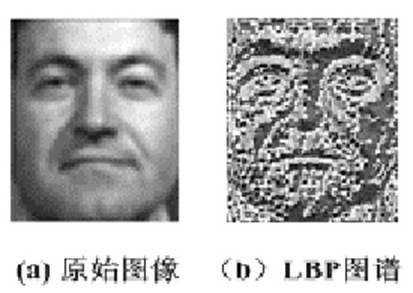LBP的应用中，如纹理分类、人脸分析等，LBP特征提取结果还是大小相同的一幅图像，一般都不将LBP图谱作为特征向量用于分类识别，而是采用LBP特征谱的统计直方图作为特征向量用于分类识别。
因为，从上面的分析我们可以看出，这个“特征”跟位置信息是紧密相关的。直接对两幅图片提取这种“特征”，并进行判别分析的话，会因为“位置没有对准”而产生很大的误差。后来，研究人员发现，可以将一幅图片划分为若干的子区域，对每个子区域内的每个像素点都提取LBP特征，然后，在每个子区域内建立LBP特征的统计直方图。如此一来，每个子区域，就可以用一个统计直方图来进行描述；整个图片就由若干个统计直方图组成；
例如：一幅100*100像素大小的图片，划分为10*10=100个子区域（可以通过多种方式来划分区域），每个子区域的大小为10*10像素；在每个子区域内的每个像素点，提取其LBP特征，然后，建立统计直方图；这样，这幅图片就有10*10个子区域，也就有了10*10个统计直方图，利用这10*10个统计直方图，就可以描述这幅图片了。之后，我们利用各种相似性度量函数，就可以判断两幅图像之间的相似性了。
3、对LBP特征向量进行提取的步骤
（1）首先将检测窗口划分为16×16的小区域（cell）；
（2）对于每个cell中的一个像素，将相邻的8个像素的灰度值与其进行比较，若周围像素值大于中心像素值，则该像素点的位置被标记为1，否则为0。这样，3*3邻域内的8个点经比较可产生8位二进制数，即得到该窗口中心像素点的LBP值；
（3）然后计算每个cell的直方图，即每个数字（假定是十进制数LBP值）出现的频率；然后对该直方图进行归一化处理。
（4）最后将得到的每个cell的统计直方图进行连接成为一个特征向量，也就是整幅图的LBP纹理特征向量；
然后便可利用SVM或者其他机器学习算法进行分类了。

转载于:https://www.cnblogs.com/pacino12134/p/11360847.html
展开全文•研究论文
• 特征提取算法探讨 点特征提取算法探讨 点特征提取算法探讨
• ## 传统特征提取算法

千次阅读 2019-02-16 18:37:42
传统特征提取算法传统特征提取算法HOG特征lbp算法 传统特征提取算法 因为以前学习过数字图像处理等传统图像处理的书籍，但是现在忘了这些算法，所以做一下笔记防止忘了。有错误的话希望大家共同交流。（前段时间怀疑...
传统特征提取算法传统特征提取算法HOG特征lbp算法
传统特征提取算法
因为以前学习过数字图像处理等传统图像处理的书籍，但是现在忘了这些算法，所以做一下笔记防止忘了。有错误的话希望大家共同交流。（前段时间怀疑自己得了抑郁症，很久没有这么忘我投入到技术中了，这种知识充实感觉希望能继续下去，后面要加入深度学习大军了~~~哈哈哈~）
HOG特征
方向梯度直方图（Histogram of Oriented Gradient, HOG）。使用梯度直方图来描述的形状特征。主要在行人检测方面。从名字可知，主要将图像中的pixel的方向变化作为特征。因为梯度变化主要是发生在物体边缘，这样就可以知道物体的大致形状。可以进行物体分类或者检测。经典的特征提取一般需要一些超参，比如窗口大小等。
算法流程从别的帖子那里拿图：
HOG特征提取方法就是将一个image（你要检测的目标或者扫描窗口）：
1）灰度化（将图像看做一个x,y,z（灰度）的三维图像）；
2）采用Gamma校正法对输入图像进行颜色空间的标准化（归一化）；目的是调节图像的对比度，降低图像局部的阴影和光照变化所造成的影响，同时可以抑制噪音的干扰；
3）计算图像每个像素的梯度（包括大小和方向）；主要是为了捕获轮廓信息，同时进一步弱化光照的干扰。
4）将图像划分成小cells（例如6*6像素/cell）；
5）统计每个cell的梯度直方图（不同梯度的个数），即可形成每个cell的descriptor；
6）将每几个cell组成一个block（例如3*3个cell/block），一个block内所有cell的特征descriptor串联起来便得到该block的HOG特征descriptor。
7）将图像image内的所有block的HOG特征descriptor串联起来就可以得到该image（你要检测的目标）的HOG特征descriptor了。这个就是最终的可供分类使用的特征向量了。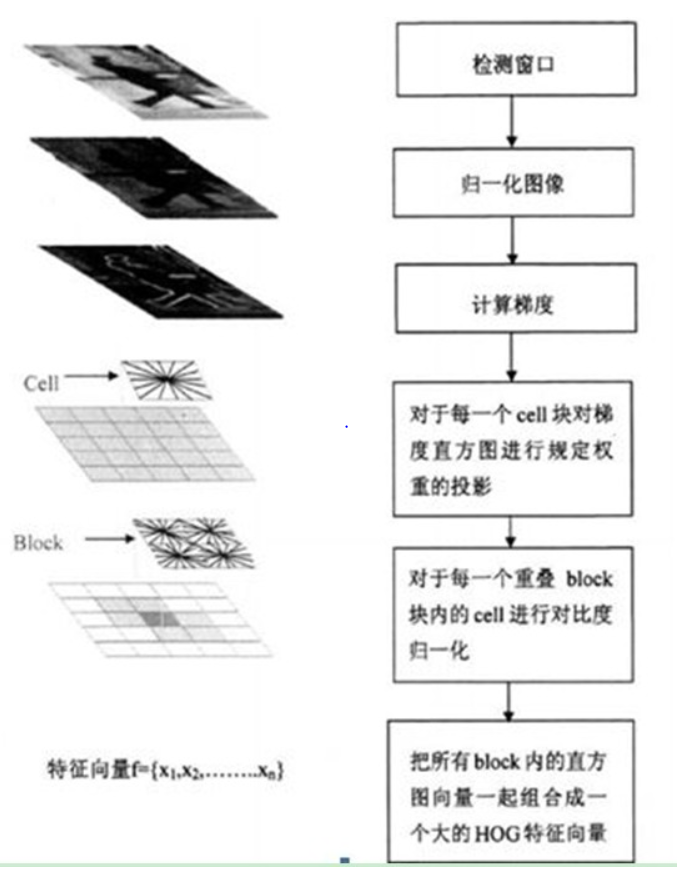计算梯度其实就是用设计好的卷积核对图像进行卷积。算法涉及的参数可以根据场景调整。
lbp算法
LBP（Local Binary Pattern，局部二值模式），反映了局部的纹理特征，跟一个像素的邻域有关。对每个像素的邻域做减法，大于用1表示，否则用0表示则产生了一个二进制数来表示这个像素的局部纹理。这个邻域其实也相当于一个window，window的形状也是可以改变的。具体算法流程可以参考这篇文章https://blog.csdn.net/q123456789098/article/details/52748918还包括了一些算法的变种。
不过经典的算法使用逐渐被深度学习模型取代，但是我觉得经典的仍然需要学习。毕竟一些传统的应用领域还在使用，或者说经典算法对于新的算法仍然具有启发意义。


展开全文图像处理
• ## 常用特征提取算法

千次阅读 2019-05-08 17:15:09
1、LBP特征提取算法 参考：http://blog.csdn.net/SoaringLee_fighting/article/details/52699381 2、HOG特征提取算法 参考：http://blog.csdn.net/abcjennifer/article/details/7365651和...
1、LBP特征提取算法
参考：http://blog.csdn.net/SoaringLee_fighting/article/details/52699381
2、HOG特征提取算法
参考：https://blog.csdn.net/u011285477/article/details/50974230
http://blog.sina.com.cn/s/blog_60e6e3d50101bkpn.html
https://blog.csdn.net/masibuaa/article/details/14056807
3、Haar特征提取算子
参考：https://blog.csdn.net/xizero00/article/details/46929261、https://www.cnblogs.com/zhehan54/p/6723956.html
4、LoG特征提取算法
参考：https://www.jianshu.com/p/2155ab92ffd3
5、Harris角点特征提取算法
参考：
6、SIFT特征提取算子
参考：https://www.jianshu.com/p/03449c6b42c4
https://blog.csdn.net/abcjennifer/article/details/7639681
7、SURF特征提取算法
http://www.cnblogs.com/tornadomeet/archive/2012/08/17/2644903.html。
8、WLD（韦伯局部描述符）
https://www.cnblogs.com/zyly/p/9570376.htm


展开全文• 在上一篇博文《OpenCV4学习笔记（38）》中记录的SIFT算法是一种包含了特征提取和描述的算法，而今天要整理记录的是分别对应于图像特征的提取和描述两个方面的FAST特征提取算法和BRIEF特征描述算法。 FAST特征提取...opencv 计算机视觉 c++
• 但是,现有的颜色特征提取算法基于单一色彩空间,颜色矩作为最常用的全局颜色特征向量,往往会因为忽略图像的空间特征导致检索错误。针对上述不足,提出了一种基于混合色彩空间分块颜色特征提取算法,并将所提取的颜色...
•研究论文
•研究论文
• 介绍了颜色矩、Hu矩、Zernike矩、小波矩等特征提取算法，改进了大小特征提取算法。针对单一特征提取算法提取特征信息不全面，不能区别对待识别样本，识别率低等问题提出了一种改进特征提取算法，该算法由上述五种...
•PYTHON
• 路面裂缝影像几何特征提取算法，李清泉，刘向龙，传统的基于人工视觉野外检测路面裂缝的方法愈来愈不能适应高速公路发展的要求，其耗人力、耗时、影响交通、危险、测量结果不一致
•人工智能
• ## 模式识别之特征提取算法

万次阅读 多人点赞 2017-12-11 22:02:24
说明：此处暂时简单介绍下各种特征提取算法，后续完善。 前言：模式识别中分类器进行分类识别时，判断的依据就是图像特征。最简单的你可以把整幅图像的像素矩阵作为图像特征进行训练和判断分类，但毕竟该特征太过...
• 由邓百川等提出的一种近红外光谱特征提取算法，A bootstrapping soft shrinkage (BOSS) approach for variable selection
• 显著性特征提取算法，和VC6.0++作为开发环境，设计实现两种有代表性的视觉显著性计算模型，并利用这种模型计算自然图像分析实验结果。
• HOG图像特征提取算法HOG简介HOG计算步骤HOG参数计算HOG提取特征效果HOG代码实现 HOG简介 HOG全称：方向梯度直方图（Histogram of Oriented Gradient），发表于2005年的CVPR，是一种图像特征提取算法，和SVM分类器...
• Word文档 三维模型特征提取算法 一特征提取需求由来 ? ? ? 虚拟装配在CAD建模领域使用广泛SolidworksPro/EUG等都有自己的零件装配程序模块但是它们相互之间并不能进行直接的数据格式转换比如Solidworks创建一个简单...
• 欢迎关注我们，选择加"星标"或“置顶”更多技术，第一时间送达特征提取算法(1)时域方法：这是比较早期的EEG信号处理方法，主要通过提取EEG的波形特征，比如振幅、方差、波峰等，对EEG信号进行分析；(2)频域方法：...
• 针对异源图像中可见光与红外图像特征提取算法进行研究，提出了一种基于边缘几何不变性特征的提取算法。采用空域滤波及灰度处理的方法对背景噪声进行处理，使用Canny算子提取目标的边缘，利用二值形态学中腐蚀与膨胀...Canny边缘...